Electron. J. Diff. Equ., Vol. 2014 (2014), No. 139, pp. 1-11.

### Eigenvalue problems with p-Laplacian operators Yan-Hsiou Cheng

Abstract: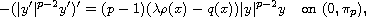where p>1 and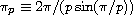. We show that if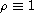and q is single-well with transition point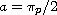, then the second Neumann eigenvalue is greater than or equal to the first Dirichlet eigenvalue; the equality holds if and only if q is constant. The same result also holds for p-Laplacian problem with single-barrierand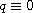. Applying these results, we extend and improve a result by  by using finitely many eigenvalues and by generalizing the string equation to p-Laplacian problem. Moreover, our results also extend a result of Huang  on the estimate of the first instability interval for Hill equation to single-well function q.Yan-Hsiou Cheng Department of Mathematics and Information Education National Taipei University of Education Taipei City 106, Taiwan email: yhcheng@tea.ntue.edu.tw